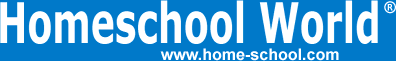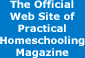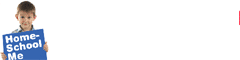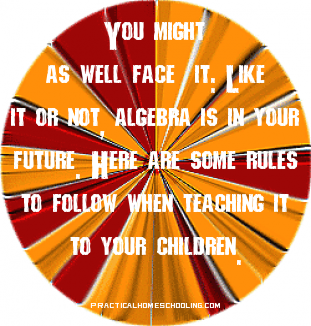Home Curriculum Catalog Articles Contests Events Groups Forum News ContactGetting Started Preschool/K Elementary Middle School High School College & Career Prep Special Needs Group Activities Research FAQs# Teaching Algebra: The Search for X

By Bill Pride
Printed in Practical Homeschooling #66, 2005.How and why to teach algebra.I was struggling through a particularly difficult concept with my daughter in her algebra the other day when in exasperation she asked, "Why do I have to study algebra anyway? What's it good for?"

The easy anwer is that it is heretical for a mathematics student to even ask such a question. Like climbing a mountain, algebra ought to be mastered just because it's there.

The truth of the matter is that algebra is inevitable for anyone who will be attending college. Everyone has to take it and pass it if they are going to make it through.

For mathematics, science, and medicine algebra is the first course in every sequence. Strong algebra skills are necessary for chemistry, and algebra is the foundation for advanced math and calculus, which are prerequisite for physics. Physics is necessary to the study of electronics.

You might as well face it: Like it or not, algebra is in your future.

Rule One: Start Early

Algebra is not difficult. A lot of algebraic ideas can be taught quite young.

As I said last issue, an algebra student has to be proficient in arithmetic. You don't want to be bogged down in addition, subtraction, multiplication, and division when you are trying to learn how to solve equations. These basic operations should be extended to decimals (numbers with digits to the right of the decimal point) also. Perhaps most importantly, your student should be excellent at doing arithmetic with fractions. Much of the way you manipulate algebraic expressions is analogous to what you do when you add, subtract, multiply, and divide fractions. Proficiency at fractions will carry over to algebra.

Beyond the four basic operations, your student should know what it means to raise a number to a power, i.e., multiplying a number by itself a certain number of times. For example, "24," read "two raised to the fourth power," is the same as "2 x 2 x 2 x 2." He should also know what a square root is.

Another important concept is the idea of "x" - the number we are trying to find. Kids are used to problems like:

4 + ___ = 8

That blank spot is where the "x" goes and you should put it in there every now and then, like this:

4 + x = 8

so your student gets comfortable with using a letter for the unknown number.

Another idea a young student can master is the idea of the number line and coordinate plane. You draw a line with an arrow on the right end, you put evenly spaced dots on the line, you label the dots with the counting numbers, and you have a number line. You can use a number line to teach counting, addition, and subtraction. You can introduce zero and extend the line to the left and show the negative numbers.

Finally you can take another number line and place it vertically so that the zeros line up. You can specify any point on the paper by its number on the horizontal line and its number on the vertical line like this: (2, 3). You find the point by going out to two on the horizontally, then up three from there. Have your student locate a series of points and connect them to draw a picture. After a little practice, graphing things in algebra will seem totally natural.

Rule Two: No Calculators

Pocket calculators first started replacing sliderules back when I was in college. We mathematics majors used to scoff at them as a crutch. As use of calculators has become a greater and greater part of elementary school and high school math curricula, our opinion of calculators has been borne out, only instead of a crutch, it appears that calculators are more like a wheelchair or maybe even a straitjacket. When immobilized by the use of calculators, the "math muscles" never develop.

Learning mathematics means learning how to manipulate numbers and mathematical symbols with your mind and a pencil and paper. You will never become proficient at math if all you learn is how to press buttons and read the answer.

Algebra, trigonometry, and calculus were all invented centuries before the microchip, and kids never "needed" calculators to do them until recently. Yet, modern algebra curricula are increasingly requiring the use of graphing calculators. This innovation was promoted by the graphing calculator manufacturers, so you don't have to be very cynical to figure out the economic motivation behind it.

A separate unit on how to use a graphing calculator might be useful after the student has learned algebra, since using calculators is now allowed when taking standardized tests such as the SAT. Because calculators are allowed, the test designers no longer formulate the problems to make sure all the answers work out to nice round numbers. Knowing how to use a calculator can save time once you know enough math to know if the answer you get is reasonable. Once you have done the math, the calculator does the arithmetic.

Rule Three: Show all Work

Math students need to get in the habit of writing out all the steps of a problem when they are solving it. A bare answer is either right or wrong. If your student doesn't write down the steps, he will either get a problem all right or all wrong. But if your student gets into the habit of writing out his problems, you can see if the answer is wrong because of a foolish arithmetic error or because the student has no notion of what he is doing. The student will learn the blessedness of partial credit and the parent will have an inkling of the thought processes that went into the student's answer and instruct him accordingly.

Rule 4: Get the Solutions

Whatever curriculum you get, get the solutions manual. I am not talking about an answer key. A solutions manual gives more than just the bottom line of the answer; it gives a step-by-step solution for the problem showing all work. Since the easiest way to learn how to do math problems is to see similar problems worked out in front of you, the solutions manual is invaluable. It allows even algebra-weak parents to check homework and award partial credit.

This "manual" does not always have to be a book. Video/DVD algebra programs such as Chalk Dust demonstrate how to do dozens of different kinds of problems for each algebra topic. The D.I.V.E. into Math CD-ROMs, based on the Saxon math text books, include short narrated animations that work out the solutions for each problem in every textbook.

Unless you are a math fanatic who just exists to solve high school algebra problems, having the solutions is a must.

Extraneous Topics

A number of topics have crept into the algebra curriculum, most of them having to do with statistics, no doubt fueled by the increasing role of statistics in our everyday lives in the U.S.A. Topics such as reading graphs; mean, median, and mode; statistical plots of data; and permutations and combinations make more sense when studied separately as a statistics unit.

Saxon texts dedicate such a lot of space to geometry in the Algebra I and II texts, you may have the impression that when you finish Algebra I and II, you have also covered geometry. This is not the case as you will see next issue when geometry is my topic.Terms of Use   Privacy Policy Copyright ©1993-2023 Home Life, Inc.